GCSE Sample Test
Paper 1
How would you like to complete the test?
Check question by question
Check the test at the end
marks

Circle the calculation that increases 400 by 7%

400 × 0.07
400 × 0.7
400 × 1.07
400 × 1.7
[1 mark]
marks

Simplify 34 × 34

38
98
316
916
[1 mark]
marks

Circle the area that is the same as 5.5 m2

550 cm2
5 500 cm2
55 000 cm2
5 500 000 cm2
[1 mark]
marks

One of these graphs is a sketch of y = 1 − 2x Which one?

Select the correct letter.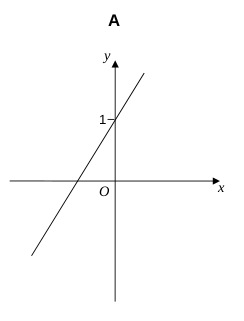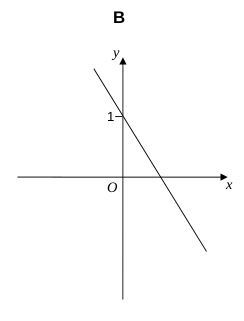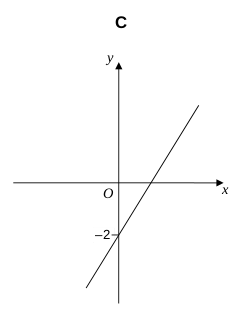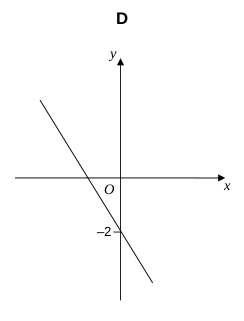[1 mark]
marks

The scatter graph shows the age and the price of 18 cars.
The cars are all the same make and model.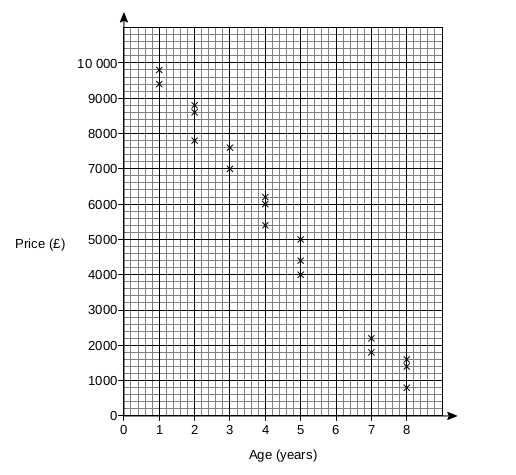Use a line of best fit to estimate the price of a 6-year old car.

£ [2 marks]
marks

Kelly is trying to work out the two values of w for which 3ww3 = 2
Her values are 1 and −1

Are her values correct?

[2 marks]
marks

Work out ×

[3 marks]
marks

Solve 5x – 2 > 3x + 11

x [2 marks]
marks

The nth term of a sequence is 2n + 1
The nth term of a different sequence is 3n − 1

Work out the three numbers that are

in both sequences

and

between 20 and 40

, , (in increasing order) [3 marks]
marks

White paint costs £2.80 per litre.
Blue paint costs £3.50 per litre.
White paint and blue paint are mixed in the ratio 3 : 2

Work out the cost of 18 litres of the mixture.

£ [4 marks]
marks

Students in a class took a spelling test.
The diagram shows information about the scores.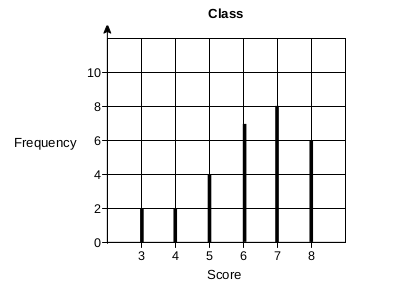Lucy is one of the 29 students in the class.
Her score was the same as the median score for her class.

Work out her score.

[2 marks]
marks

ABCH is a square.
HCFG is a rectangle.
CDEF is a square.

They are joined to make an L-shape.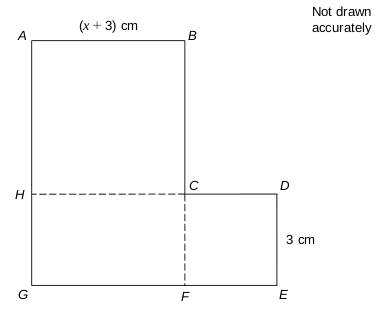Show that the total area of the L-shape, in cm2, is of the from ax2 + bx + c and give the values of a, b, and c

a =

b =

c =

[4 marks]
marks

Here are sketches of four triangles.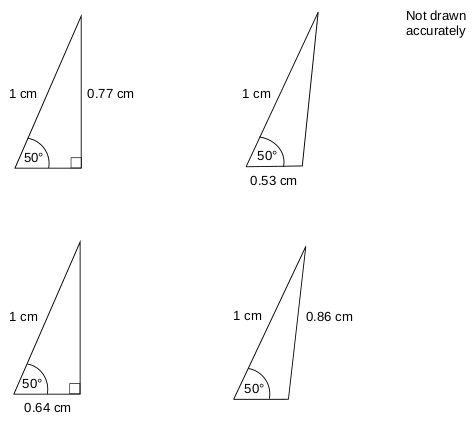In each triangle

the longest side is exactly 1 cm

the other length is given to 2 decimal places.

(a)

Circle the value of cos 50° to 2 decimal places.

0.77
0.53
0.64
0.86
[1 mark]
(b)

Work out the value of x.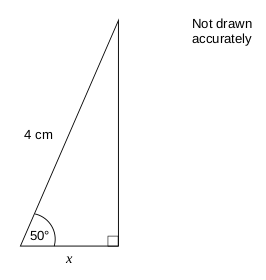x = cm [2 marks]
marks

A prime number between 300 and 450 is chosen at random. The table shows the probability that the number lies in different ranges.

 Prime number, n Probability 300 ≤ n < 330 0.16 330 ≤ n < 360 0.24 360 ≤ n < 390 x 390 ≤ n < 420 0.16 420 ≤ n < 450 0.24
(a)

Work out the value of x.

[2 marks]
(b)

Work out the probability that the prime number is greater than 390

p = [1 mark]
(c)

There are four prime numbers between 300 and 330
How many prime numbers are there between 300 and 450?

prime numbers [2 marks]
marks

a × 104 + a × 102 = 24 240 where a is a number.

Work out a × 104a × 102

× 10 [2 marks]
marks

AB, CD and YZ are straight lines.
All angles are in degrees.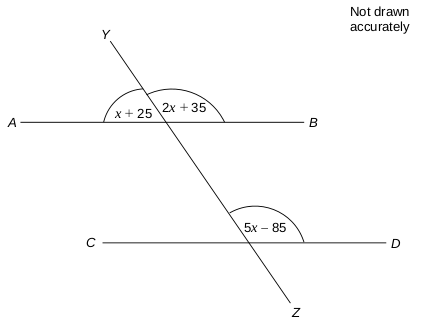(a)

Find the value of x.

x = ° [2 marks]
(b)

Which of these options is true:

AB is not parallel to CD
AB is parallel to CD
You cannot tell whether AB is parallel or not parallel to CD
[2 marks]
marks

To complete a task in 15 days a company needs

4 people each working for 8 hours per day.

The company decides to have

5 people each working for 6 hours per day.

Assume that each person works at the same rate.

How many days will the task take to complete?

days [3 marks]
marks

In this question all dimensions are in centimetres.

A solid has uniform cross section.
The cross section is a rectangle and a semicircle joined together.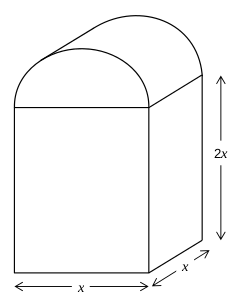Work out an expression, in cm3, for the total volume of the solid.

Write your expression in the form ax3 + πx3 where a and b are integers.

x3 + πx3 [4 marks]
marks

Show that 12 cos 30° − 2 tan 60° can be written in the form where k is an integer.

What is the value of k?

k = [3 marks]
marks

On Friday, Greg takes part in a long jump competition.

He has to jump at least 7.5 metres to qualify for the final on Saturday.

He has up to three jumps to qualify.

If he jumps at least 7.5 metres he does not jump again on Friday.

Each time Greg jumps, the probability he jumps at least 7.5 metres is 0.8

Assume each jump is independent.

(a)

Complete the tree diagram.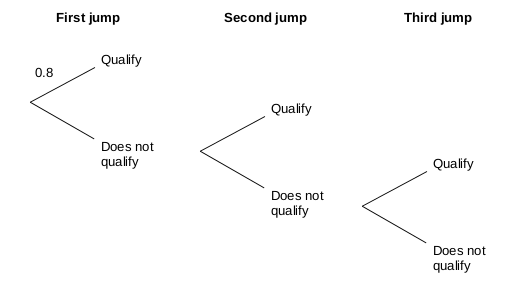[2 marks]
(b)

Work out the probability that he does not need the third jump to qualify.

p = [2 marks]
marks

A, B and C are points on a circle.

BC bisects angle ABQ.

PBQ is a tangent to the circle.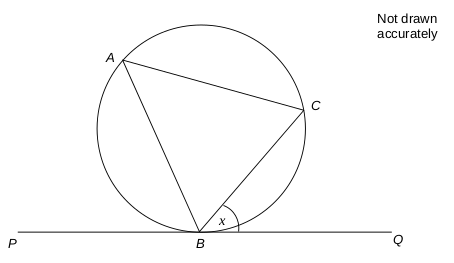Angle CBQ = x

What is the value of angle ACB in terms of x?

ACB = [3 marks]
marks

Steph is solving a problem.

Cube A has a surface area of 150 cm2
Cube B has sides half the length of cube A

What is the volume of cube B?

V = cm3 [2 marks]
marks

Square OABC is drawn on a centimetre grid.

O is (0, 0) A is (2, 0) B is (2, 2) C is (0, 2)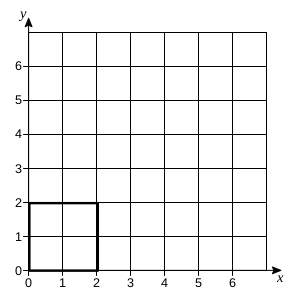(a)

OABC is translated by the vector

Circle the number of invariant points on the perimeter of the square.

0
1
2
4
[1 mark]
(b)

OABC is enlarged, scale factor 2, centre (0, 0)

Circle the number of invariant points on the perimeter of the square.

0
1
2
4
[1 mark]
(c)

OABC is reflected in the line y = x

Circle the number of invariant points on the perimeter of the square.

0
1
2
4
[1 mark]
marks

Here is the velocity-time graph of a car for 50 seconds.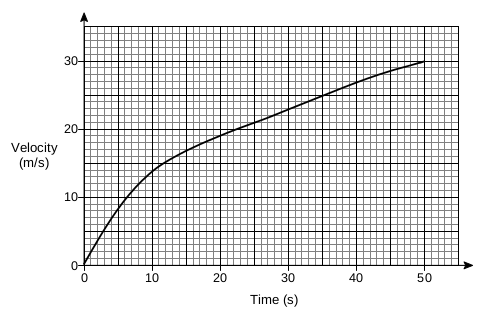(a)

Work out the average acceleration during the 50 seconds.

[2 marks]
(b)

Estimate the time during the 50 seconds when

the instantaneous acceleration = the average acceleration

seconds [2 marks]
marks

f(x) = 2x + c

g(x) = cx + 5

fg(x) = 6x + d

c and d are constants.

Work out the value of d.

d = [3 marks]
marks

Rationalise the denominator and simplify

[2 marks]
marks

Convert 0.172 to a fraction in its lowest terms.

[3 marks]
marks

The diagram shows the circle x2 + y2 = 10

P lies on the circle and has x-coordinate 1

The tangent at P intersects the x-axis at Q.

Work out the coordinates of Q.

Q(,) [5 marks]
Test check
Total: - of marks